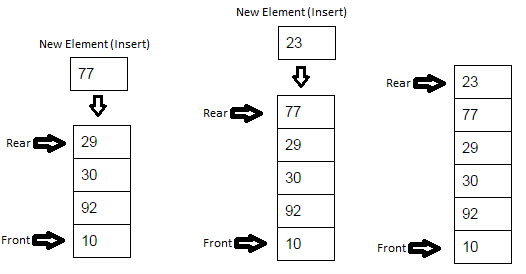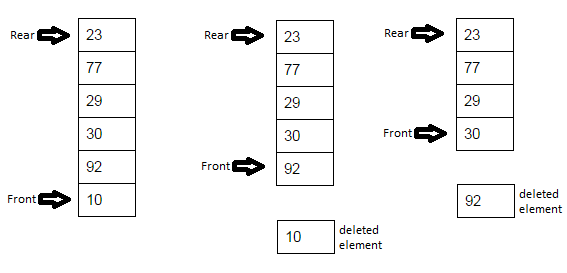### Queues implementation in java

In this Data structures tutorial we will learn what is Queues in java with example and program. We will learn how to implement your own Queues in java. We will learn how to insert and remove element from Queues in java.

Queue is Collection of entities or elements in which >
Addition of element is done at REAR.
Removal of element is done at FRONT.
Queue follows FIFO (First in first out) - means  the first element added to the queue will be the first one to be removed.

Let’s see a queue of people-
>people will join Queue at rear (Addition of element)
>people will leave Queue from front (Removal of element)Logic explanation of Queues in java >

Inserting element in Queue in java>Removing element from Queue in java>Full Program/Example of Queues implementation in java, insert and remove element from Queues in java >
 /** *Exception to indicate that Queue is full. */ class QueueFullException extends RuntimeException {       public QueueFullException(){        super();    }        public QueueFullException(String message){        super(message);    }     } /** *Exception to indicate that Queue is empty. */ class QueueEmptyException extends RuntimeException {       public QueueEmptyException(){        super();    }        public QueueEmptyException(String message){        super(message);    }     } /** Copyright (c), AnkitMittal JavaMadeSoEasy.com */ /** * Queue class */ class Queue {       private int[] queueAr;    private int size; //Size of Queue       private int rear; //elements will be added at rear.    private int front;   //elements will be removed from front    private int number;  //holds number of elements in Queue, initialized with 0 by default    /**    * Constructor    */    public Queue(int size) {           this.size = size;           queueAr = new int[this.size];                   rear = 0;           front = 0;    }    /**    * Insert element at rear in Queue    */    public void insert(int value){                     if(isFull()){                  throw new QueueFullException("Cannot insert "+value+", Queue is full");           }                    if (rear == size ) // means we are at last position (deal with wrapAround)                  rear = 0;           queueAr[rear++] = value; // Insert element and increment rear           number++; //increase number of elements in Queue    }       /**    * Removes elements from front of Queue    */    public int remove(){           if(isEmpty()){                  throw new QueueEmptyException("Queue is empty");           }                     int deletedValue = queueAr[front++]; // get value at front and than increment front           if (front == size) // deal with wrapAround                  front = 0;           number--; //reduce number of elements in Queue           return deletedValue;    }    /**    * @return true if Queue is empty    */    public boolean isEmpty() {           return (number == 0);    }       /**    * @return true if Queue is full    */    public boolean isFull() {           return (number == size);    }    } /** * QueueExample - Main class */ public class QueueExample {    public static void main(String[] args) {           Queue queue = new Queue(10); // queue holds 10 elements                     queue.insert(31);           queue.insert(49);           queue.remove();           queue.remove();           queue.insert(90);           queue.insert(81);           queue.insert(72);           queue.insert(22); // At this point we got to deal with wrapAround, because rear must be pointing to last position.                     System.out.print("Deleted elements from queue: ");           System.out.print(queue.remove()+ " ");           System.out.print(queue.remove()+ " ");           System.out.print(queue.remove()+ " ");           System.out.print(queue.remove()+ " ");              } } /** OUTPUT Deleted elements from queue: 90 81 72 22 */
If we analyze output queue example, we will find that- first element added to the queue was the first one to be removed in java.

Complexity of Queues in java>

Insert - O(1) [as we insert element at Rear of queue] in java
Remove - O(1) [as we remove element from front of queue] in java

So in this Data structures tutorial we learned what is Queues in java with example and program. We will learn how to implement your own Queues in java. We learned how to insert and remove element from Queues in java.

Having any doubt? or you you liked the tutorial! Please comment in below section.

1) Stacks, Queues in Data Structures in java

2) Single LinkedList implementations in Data Structures in java:-

3) Doubly LinkedList implementations in Data Structures in java:-

5) Some of the tricky and interesting Single LinkedList implementations in Data Structures in java

6) Binary tree tutorial in java >